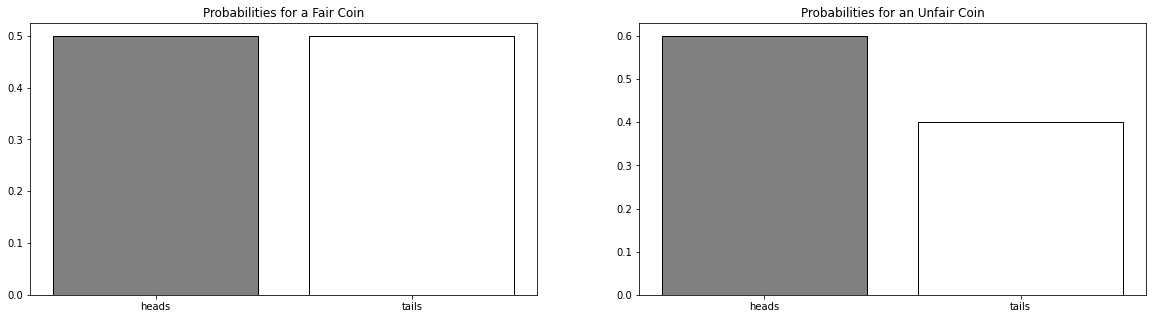# Introduction to Probability¶

The probability of an event is a number between 0 and 1, where, roughly speaking, 0 indicates impossibility of the event and 1 indicates certainty. The higher the probability of an event, the more likely it is that the event will occur. A simple example is the tossing of a fair (unbiased) coin. Since the coin is fair, the two outcomes ("heads" and "tails") are both equally probable; the probability of "heads" equals the probability of "tails"; and since no other outcomes are possible, the probability of either "heads" or "tails" is 1/2 (which could also be written as 0.5 or 50%). --- Source

In [ ]:
import matplotlib.pyplot as plt
import numpy as np
import pandas as pd


## Example: Tossing Coins¶

Consider the example of tossing a fair coin to root our vocabulary and symbols. We will say the probability of getting a head is the total ways for this event to happen divided by the number of all possible outcomes.

EXAMPLE I: A Fair Coin

With one fair coin, we have outcomes either heads or tails. The outcome of interest is either a heads or tails. We would say the probability of each is $\frac{1}{2}$:

$$P(\text{head}) = \frac{1}{2} \quad P(\text{tails}) = \frac{1}{2}$$

Note that these probabilities sum to 1.

EXAMPLE II: An Unfair Coin

An example of an unfair coin would be anything that does not have equal probability. For example:

$$P(\text{head}) = \frac{6}{10} \quad P(\text{tails}) = \frac{4}{10}$$
In [ ]:
import scipy.stats as stats
fig, ax = plt.subplots(1, 2, figsize = (20, 5))
fair_coin = stats.bernoulli(.5).pmf([0, 1])
ax.bar(['heads', 'tails'], fair_coin, color=['gray', 'white'], edgecolor = 'black')
ax.set_title('Probabilities for a Fair Coin');

unfair_coin = stats.bernoulli(.4).pmf([0, 1])
ax.bar(['heads', 'tails'], unfair_coin, color = ['gray', 'white'], edgecolor = 'black')
ax.set_title('Probabilities for an Unfair Coin');### Probability Mass Function¶

We can describe this kind of event as a function. Specifically, a situation in which there are two possible outcomes with a probability attached to each. This is called a bernoulli distribution, and the functional form is:

$$f(k;p)=pk+(1-p)(1-k)\quad {\text{for }}k\in \{0,1\}$$

In our example of an unfair coin, if we consider heads as 0 and tails as 1 ($k$), we would have:

$$f(0, .4) = .4*0 + (1 - .4)(1 - 0)$$

which equals 0.6. Similarly, the probability of a tails ($k = 0$):

$$f(1, .4) = .4 * 1 + (1 - 1)(1 - 1)$$

which equals 0.4.

In [ ]:
def bernoulli(k, p):
return p*k + (1-p)*(1 - k)

In [ ]:
bernoulli(0, 0.4)

Out[ ]:
0.6
In [ ]:
bernoulli(1, 0.4)

Out[ ]:
0.4

### PROBLEMS¶

1. Suppose a bag contains 8 Red Marbles and 3 Blue Marbles.
• What is the probability of choosing a red ball from a random draw?
• A Blue Ball?
2. Suppose a bag contain 4 Red Marbles, 7 Yellow Marbles, and 2 Blue Marbles.
• What is the probability of selecting a red or yellow marble?
• What is the probability of selecting a yellow or blue marble?
• How many ways can you select two colors of marbles from the bag?
3. Suppose a bag contains 10 Red and 7 Blue marbles.
• Suppose you select one marble from the bag, what is the probability this is red?
• Suppose you select a blue marble, place the marble back in the bag and select another marble. What is the probability the second marble is blue?
• Suppose you select a blue marble, do not replace the marble the bag and select another marble. What is the probability the second marble is blue?
In [ ]:


In [ ]:


In [ ]:


In [ ]: